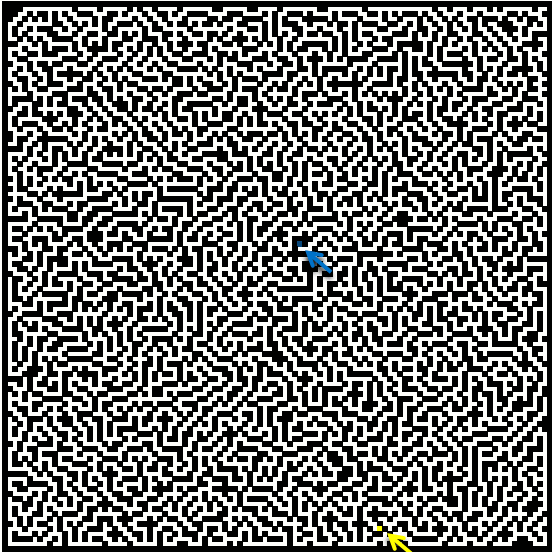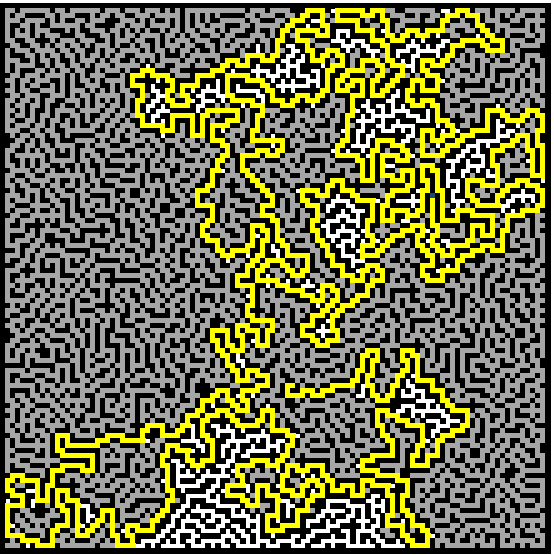Author: Oscar Cronquist Article last updated on August 11, 2017

My last post demonstrated how to build a random maze. You might remember that there was only one path between the start point and the end point.

This post shows you how to find the path between the start and end cell. The macro moves randomly around the maze and remembers where it has been. As soon as it finds the end coordinates, it stops. Conditional formatting highlights the found path yellow. You can sometimes see the macro going back and trying a new path.

I have inserted two arrows to make it easier for you finding the start point and the end point.### Show visited cells

It is also possible to highlight visited cells, change line 23:
Range("B2:DG111").Cells(loc(0, UBound(loc, 2)), loc(1, UBound(loc, 2))) = ""
to
Range("B2:DG111").Cells(loc(0, UBound(loc, 2)), loc(1, UBound(loc, 2))) = "V"

Also create a new conditional formatting rule:
=B2="V"
Apply the rule to cell range =\$B\$2:\$DG\$111. I picked a grey formatting color.It has been almost all over the maze, in this example.

### VBA Code

```Sub FindPath()

Dim loc() As Variant
ReDim loc(1, 0)
Application.ScreenUpdating = False
Set Sp = Cells.Find("S", LookIn:=xlValues, LookAt:=xlWhole, SearchOrder:=xlByRows, SearchDirection:=xlNext)
loc(1, 0) = Sp.Column - 1
loc(0, 0) = Sp.Row - 1
Set Ep = Cells.Find("E", LookIn:=xlValues, LookAt:=xlWhole, SearchOrder:=xlByRows, SearchDirection:=xlNext)
Ec = Ep.Column - 1
Er = Ep.Row - 1
mtx = Range("B2:DG111").Value
mtx(Er, Ec) = ""
Counter = 0
Do
chk = False
If mtx(loc(0, UBound(loc, 2)) + 1, loc(1, UBound(loc, 2))) = "" Then chk = True
If mtx(loc(0, UBound(loc, 2)) - 1, loc(1, UBound(loc, 2))) = "" Then chk = True
If mtx(loc(0, UBound(loc, 2)), loc(1, UBound(loc, 2)) + 1) = "" Then chk = True
If mtx(loc(0, UBound(loc, 2)), loc(1, UBound(loc, 2)) - 1) = "" Then chk = True
If chk = False Then
If loc(0, UBound(loc, 2)) = Sp.Row - 1 And loc(1, UBound(loc, 2)) = Sp.Column - 1 Then
Else
Range("B2:DG111").Cells(loc(0, UBound(loc, 2)), loc(1, UBound(loc, 2))) = ""
ReDim Preserve loc(UBound(loc, 1), UBound(loc, 2) - 1)
If Tcounter < Counter Then
Tcounter = Counter
Exr = loc(0, UBound(loc, 2) - 1)
Exc = loc(1, UBound(loc, 2) - 1)
End If
Counter = Counter - 1
End If
Else
ReDim Preserve loc(UBound(loc, 1), UBound(loc, 2) + 1)
c = False
Do Until c = True
rrand = Int(Rnd * 4)
Select Case rrand
Case 0
If mtx(loc(0, UBound(loc, 2) - 1) + 1, loc(1, UBound(loc, 2) - 1)) = "" Then
loc(0, UBound(loc, 2)) = loc(0, UBound(loc, 2) - 1) + 1
loc(1, UBound(loc, 2)) = loc(1, UBound(loc, 2) - 1)
c = True
End If
Case 1
If mtx(loc(0, UBound(loc, 2) - 1) - 1, loc(1, UBound(loc, 2) - 1)) = "" Then
loc(0, UBound(loc, 2)) = loc(0, UBound(loc, 2) - 1) - 1
loc(1, UBound(loc, 2)) = loc(1, UBound(loc, 2) - 1)
c = True
End If
Case 2
If mtx(loc(0, UBound(loc, 2) - 1), loc(1, UBound(loc, 2) - 1) + 1) = "" Then
loc(0, UBound(loc, 2)) = loc(0, UBound(loc, 2) - 1)
loc(1, UBound(loc, 2)) = loc(1, UBound(loc, 2) - 1) + 1
c = True
End If
Case 3
If mtx(loc(0, UBound(loc, 2) - 1), loc(1, UBound(loc, 2) - 1) - 1) = "" Then
loc(0, UBound(loc, 2)) = loc(0, UBound(loc, 2) - 1)
loc(1, UBound(loc, 2)) = loc(1, UBound(loc, 2) - 1) - 1
c = True
End If
End Select
Loop
mtx(loc(0, UBound(loc, 2)), loc(1, UBound(loc, 2))) = "S"
Range("B2:DG111").Cells(loc(0, UBound(loc, 2)), loc(1, UBound(loc, 2))) = "S"
Counter = Counter + 1
End If
k = k + 1
If (k / 500) - Int(k / 500) = 0 Then
Application.ScreenUpdating = True
Application.ScreenUpdating = False
End If
Loop Until loc(0, UBound(loc, 2)) = Er And loc(1, UBound(loc, 2)) = Ec
Range("B2:DG111").Cells(Er, Ec) = "E"
Application.ScreenUpdating = True
End Sub
```